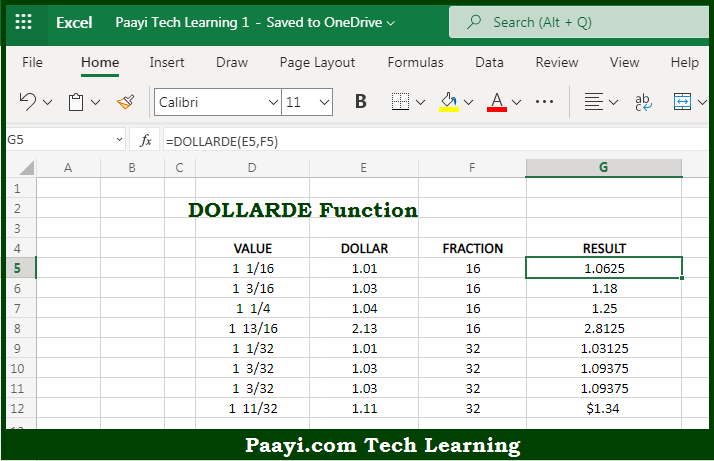# Learn How to Use Microsoft Excel DOLLARDE Function

Written by | 0 Comments | 512 Views

In this article, you will learn how to use the Microsoft Excel DOLLARDE function and its prime function in Microsoft Excel. You will also get to know the Microsoft Excel DOLLARDE function return value and syntax with the help of some examples.

Microsoft Excel DOLLARDE Function

The main purpose of the Microsoft Excel DOLLARDE function is to convert dollar prices as fractions to decimals. That implies, with the help of DOLLARDE function you can able to convert a dollar price entered with a special notation to a dollar price displayed as a decimal number.  It should be noted that the DOLLARFR function does the opposite conversion. So, with the help of DOLLARDE function, you can able to convert the dollar price a a fraction to a decimal.

Return Value of DOLLARDE Function

The return value will be the equivalent decimal number.

Syntax of DOLLARDE Function

=DOLLARDE(fractional-dollar, fraction)

Where the arguments:

• fractional-dollar: This is the dollar component in special fractional notation.
• fraction: This is the denominator in the fractional unit. 8 = 1/8, 16 = 1/16, 32 = 1/32, etc.

How to Use Microsoft Excel DOLLARDE Function?So we know that Microsoft Excel DOLLARDE function you can able to convert dollar price as a fraction to decimal. That implies, with the help of DOLLARDE function you can able to convert a dollar price entered with a special notation to a dollar price displayed as a decimal number.  It should be noted that the DOLLARFR function does the opposite conversion. So, with the help of DOLLARDE function, you can able to convert the dollar price a fraction to a decimal.